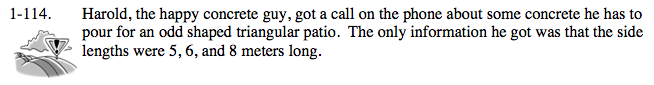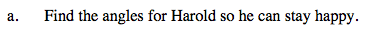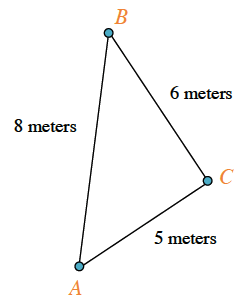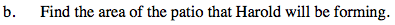### Home > PC > Chapter 1 > Lesson 1.3.2 > Problem1-114

1-114.Draw and label a diagram.

Since no angle measures are given, what formula should be used?

$6^2=5^2+8^2-2(5)(8)\cos{A}$

$36=89-80\cos{A}$

$-53 = -80\cos{A}$

$\frac{53}{80}=\cos{A}$

Law of Cosines
a² = b² + c² - 2bc(cos A)Use the Law of Sines to find another angle.

$\frac{a}{\text{Sin}A} = \frac{b}{\text{Sin}B} = \frac{c}{\text{Sin}C}$Draw a segment from angle C ⊥ to side AB.
Label the intersection point E.

Using the sine function, calculate the height, EC.

Determine the area of ΔABC by calculating half of the base (8) times the height (EC).

14.98 sq. meters